×
Oct/01/2022: Apple Inc (California, US) is looking for talents with 3GPP RAN2 experience and a good physical layer understanding. Contact Me if you are interested.

# Code block segmentation and code block CRC attachmentV15.9.0

### Polar coding

The input bit sequence to the code block segmentation is denoted by a0, a1, a2, a3,...,aA-1, where A > 0.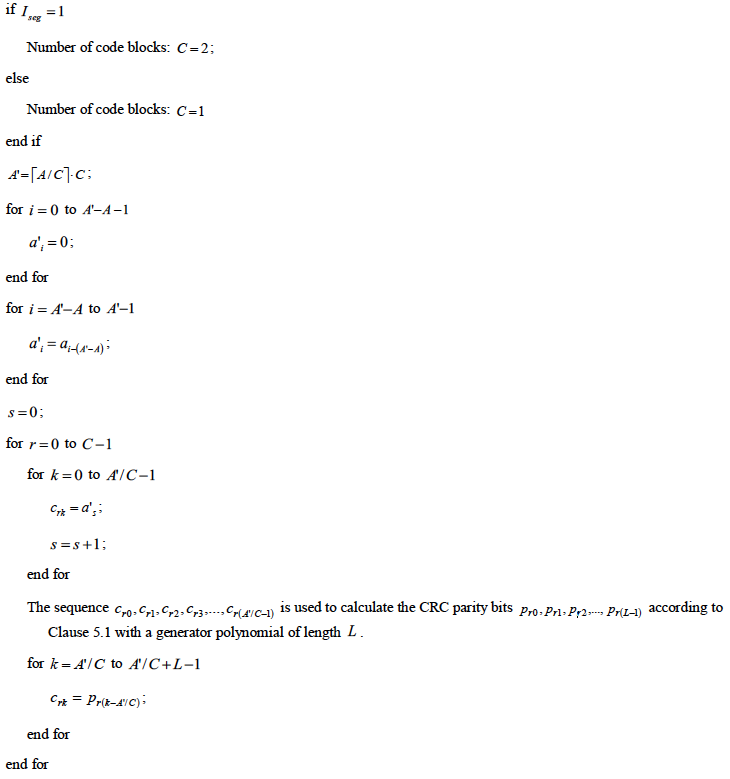The value of A is no larger than 1706.

What it means: Input bits can be divided into one or two code blocks. If odd number of input bits are to be divided into two code blocks, a 0 is prepended before the input data. Each code block has its own CRC bits.

### Low density parity check coding

The input bit sequence to the code block segmentation is denoted by b0, b1, b2, b3,..., bB-1, where B > 0. If B is larger than the maximum code block size Kcb, segmentation of the input bit sequence is performed and an additional CRC sequence of L = 24 bits is attached to each code block.

• For LDPC base graph 1, the maximum code block size is: Kcb = 8448.
• For LDPC base graph 2, the maximum code block size is: Kcb = 3840.

Figure below shows the CB segmentation for LDPC code, when input data size is larger than kcb.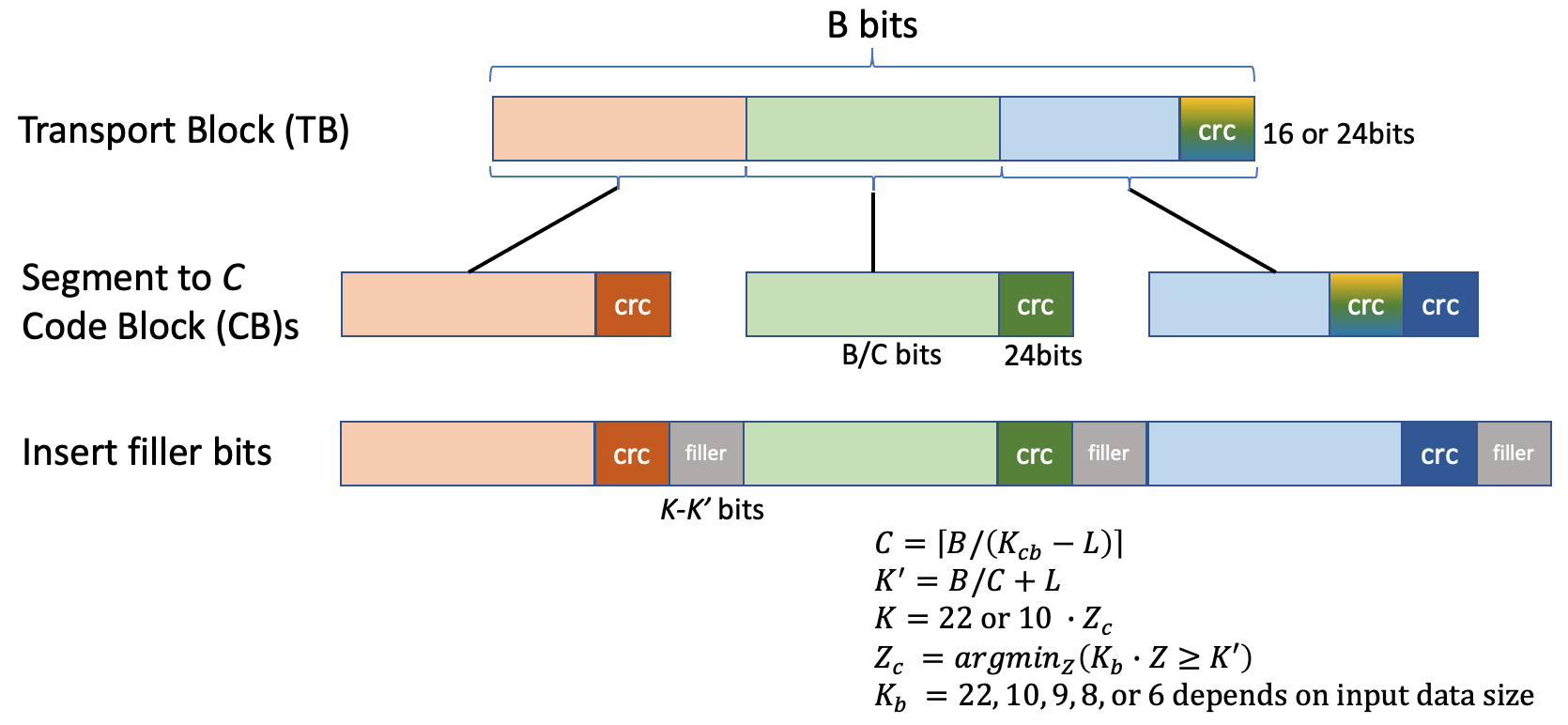Total number of code blocks C is determined by: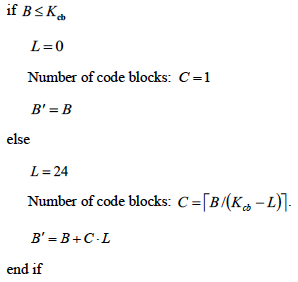What it means: Input bits are divided into multiple code blocks not exceeding 8448 bits for BG1 or 3840 bits for BG2. If there is only one code block, no extra CRC is needed. Otherwise, length-24 CRC (generation polynomial CRC24B) will be performed for each code block, and hence the data size is increased by 24 * number of code blocks.

The bits output from code block segmentation are denoted by cr0, cr1, cr2, cr3,...,cr(Kr-1), where 0 ≤ r < C is the code block number, and Kr = K is the number of bits for the code block number r.

The number of bits K in each code block is calculated as: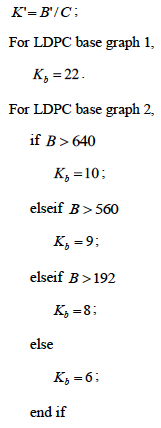find the minimum value of Z in all sets of lifting sizes in Table 5.3.2-1, denoted as Zc, such that Kb·Zc≥K', and set K = 22Zc for LDPC base graph 1 and K = 10Zc for LDPC base graph 2;

What it means:

Lifting size is used to identify an allowed code block size, it takes the value of 2, 3, 5, 7, 9, 11, 13, 15 multplied by 2n. Maximum value of the lifting size is 8448/22 = 384, i.e. 3 * 27.

Once the lifting size Zc is determined, the code block size can be calculated, which is 22*Zc for BG1 and 10*Zc for BG2.

The bit sequence crk is calculated as: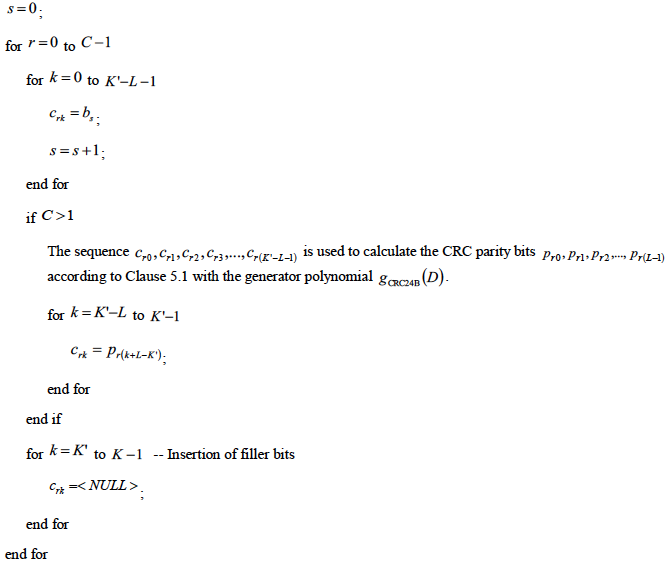What it means:

for each code block, the first K' - L bits are taken sequentially from the input data. These bits are followed by 24 CRC parity bits if code block segmentation is needed (C ≥ 1). Filler bits are appended in the end to ensure each code block has fixed size of K.